# Euler Equation

## Various Forms

### Lagrangian Representation

Among the principal governing equations we have included the

Lagrangian Representation
of the Euler Equation,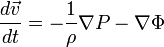$\frac{d\vec{v}}{dt} = - \frac{1}{\rho} \nabla P - \nabla \Phi$

Multiplying this equation through by the mass density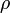$~\rho$ produces the relation,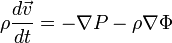$\rho\frac{d\vec{v}}{dt} = - \nabla P - \rho\nabla \Phi$ ,

which may be rewritten as,$\frac{d(\rho\vec{v})}{dt}- \vec{v}\frac{d\rho}{dt} = - \nabla P - \rho\nabla \Phi$ .

Combining this with the Standard Lagrangian Representation of the Continuity Equation, we derive,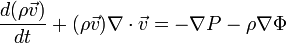$\frac{d(\rho\vec{v})}{dt}+ (\rho\vec{v})\nabla\cdot\vec{v} = - \nabla P - \rho\nabla \Phi$ .

### Eulerian Representation

By replacing the so-called Lagrangian (or "material") time derivative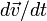$d\vec{v}/dt$ in the first expression by its Eulerian counterpart (see the linked Wikipedia discussion, and references therein, to understand how the Lagrangian and Eulerian descriptions of fluid motion differ from one another conceptually as well as how to mathematically transform from one description to the other), we directly obtain the

Eulerian Representation
of the Euler Equation,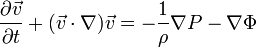$~\frac{\partial\vec{v}}{\partial t} + (\vec{v}\cdot \nabla) \vec{v}= - \frac{1}{\rho} \nabla P - \nabla \Phi$

As was done above in the context of the Lagrangian representation of the Euler equation, we can multiply this expression through by$~\rho$ and combine it with the continuity equation to derive what is commonly referred to as the,

Conservative Form
of the Euler Equation,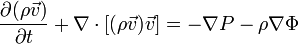$~\frac{\partial(\rho\vec{v})}{\partial t} + \nabla\cdot [(\rho\vec{v})\vec{v}]= - \nabla P - \rho \nabla \Phi$

The second term on the left-hand-side of this last expression represents the divergence of the "dyadic product" of the vector momentum density ($~\rho$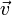$~\vec{v}$) and the velocity vector$~\vec{v}$ and is sometimes written as,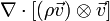$\nabla\cdot [(\rho \vec{v}) \otimes \vec{v}]$; it may help the reader to see this term written out in component form or subscript notation, as presented in the following linked Wikipedia discussion.

## Time-independent Behavior

### Lagrangian Frame of Reference (hydrostatic balance)

If you are riding along with a fluid element — viewing the system from a Lagrangian frame of reference — the velocity$~\vec{v}$ of your fluid element will, by definition, remain unchanged over time if,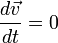$\frac{d\vec{v}}{dt} = 0$ .

From the above "Lagrangian Representation" of the Euler equation, this also leads to what is often referred to in discussions of stellar structure as the statement of,

Hydrostatic Balance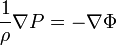$\frac{1}{\rho} \nabla P = - \nabla \Phi$ .

That is to say, every fluid element within a star will experience no net acceleration if the gradient of the pressure balances the gradient in the gravitational field throughout the star.

### Eulerian Frame of Reference (steady-state flow field)

On the other hand, if you are standing at a fixed location in your coordinate frame watching the fluid flow past you — viewing the system from an Eulerian frame of reference — the velocity of the fluid at your location in space will, by definition, always be the same if,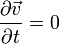$\frac{\partial\vec{v}}{\partial t} = 0$ .

From the above "Eulerian Representation" of the Euler equation, this condition also implies that a steady-state velocity field must obey the relation,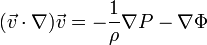$(\vec{v}\cdot \nabla) \vec{v}= - \frac{1}{\rho} \nabla P - \nabla \Phi$ .

Or, a steady-state momentum density field must obey the relation,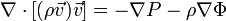$\nabla\cdot [(\rho\vec{v})\vec{v}]= - \nabla P - \rho \nabla \Phi$

 © 2014 - 2020 by Joel E. Tohline |   H_Book Home   |   YouTube   | Appendices: | Equations | Variables | References | Ramblings | Images | myphys.lsu | ADS |